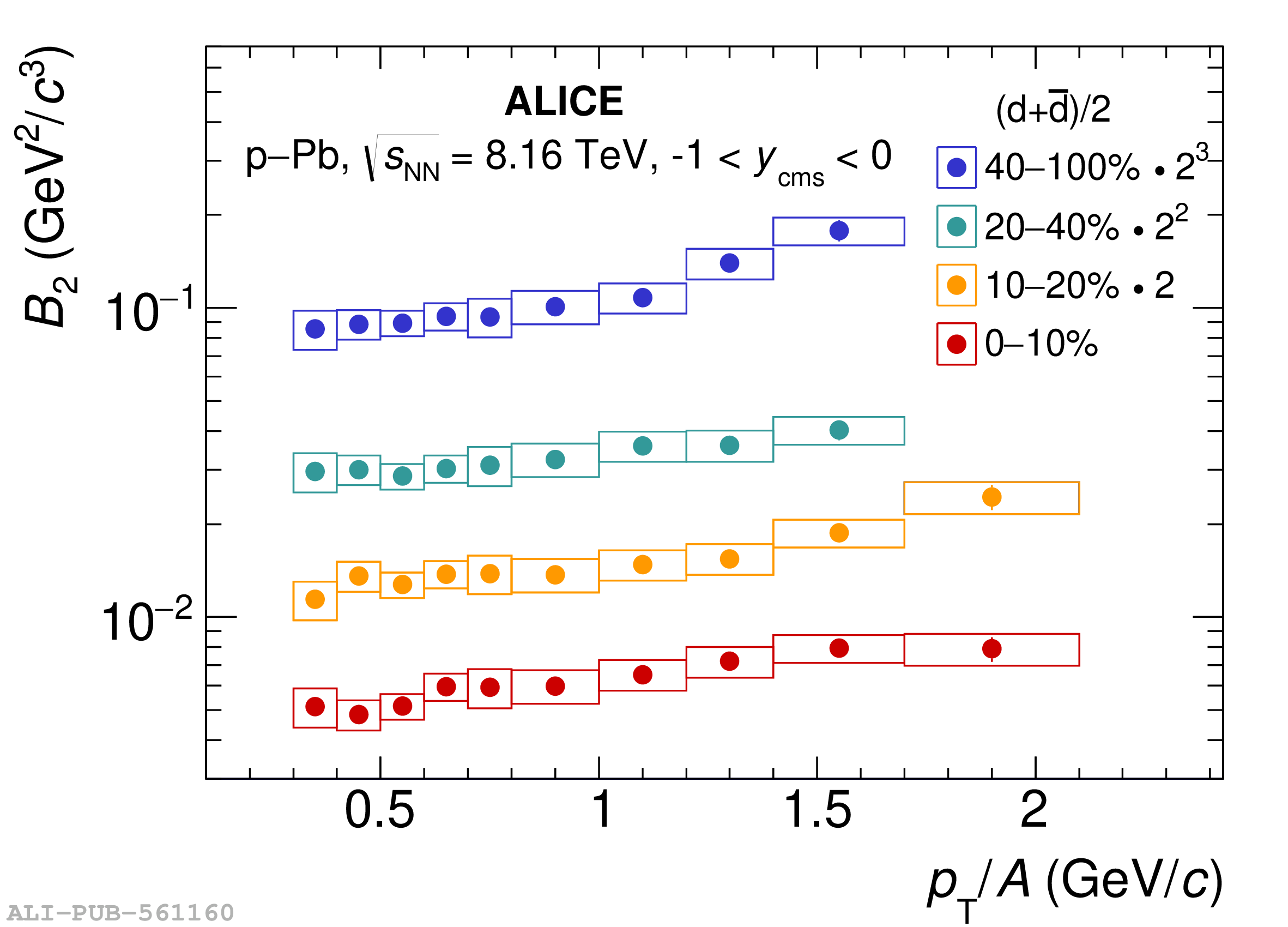# Figure 2

 Coalescence parameters $B_2$ (left panel) and $B_3$ (right panel) as a function of $p_{\mathrm T}$/$A$, measured for deuterons and $^3$He, respectively. Statistical uncertainties are represented as vertical lines whereas boxes represent the systematic ones.